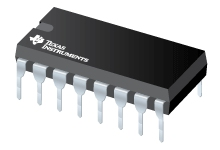CD4089B CMOS Binary Rate Multiplier | TI.com

# CD4089B ACTIVE This product has been released to the market and is available for purchase. For some products, newer alternatives may be available. CMOS Binary Rate MultiplierDatasheet

• Description
• Features
• Diagram
• Parametrics

## Description

CD4089B is a low-power 4-bit digital rate multiplier that provides an output pulse rate that is the clock-input-pulse rate multiplied by 1/16 times the binary input. For example, when the binary input number is 13, there will be 13 output pulses for every 16 input pulses. This device may be used in conjunction with an up/down counter and control logic used to perform arithmetic operations (adds, subtract, divide, raise to a power), solve algebraic and differential equations, generate natural logarithms and trigometric functions, A/D and D/A conversions, and frequency division.

For words of more than 4-bits, CD4089B devices may be cascaded in two different mode: an Add mode and a Multiply mode (see Figs. 14 and 15). In the Add mode some of the gaps left by the more significant unit at the count of 15 are filled in by the less significant units. For example, when two units are cascaded in the Add mode and programmed to 11 and 13, respectively, the more significant unit will have 11 output pulses for every 16 input pulses and the other unit will have 13 output pulses for every 256 input pulses for a total of

 11 13 189 — + — = — 16 256 256

In the Multiply mode the fraction programmed into the first rate multipliers multiplied by the fraction programmed into the second multiplier. Thus the output rate will be

 11 13 143 — × — = — 16 16 256

The CD4089B has an internal synchronous 4-bit counter which, together with one of the four binary input bits, produces pulse trains as shown in Fig. 2.

If more than one binary input bit is high, the resulting pulse train a combination of the separate pulse trains as shown in Fig. 2.

The CD4089B types are supplied in 16-lead hermetic dual-in-line ceramic packages (F3A suffix), 16-lead dual-in-line plastic packages (E suffix), 16-lead small-outline packages (NSR suffix), and 16-lead thin shrink small-outline packages (PW and PWR suffixes).

## Features

• Cascadable in multiples of 4-bits
• Set to "15" input and "15" detect output
• 100% tested for quiescent current at 20V
• 5-V, 10-V, and 15-V parametric ratings
• Standardized, symmetrical output characteristics
• Maximum input current of 1 µA at 18 V over full package-temperature range; 100nA at 18 V and 25°C
• Noise margin (full package-temperature range) =
1 V at VDD = 5 V
2 V at VDD = 10 V
2.5 V at VDD = 15 V
• Meets all requirements of JEDEC Tentative Standard No. 13B, "Standard Specifications for Description of ’B’ Series CMOS Devices"
• Applications:
• Numerical control
• Instrumentation
• Digital filtering
• Frequency synthesis